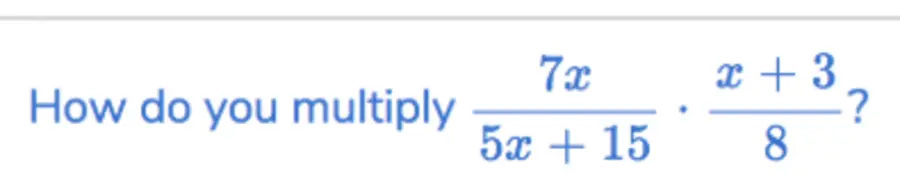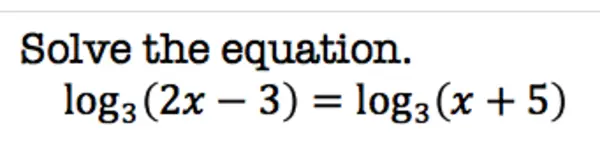# Mathpix now supports text OCR

Our customers frequently encounter images that contains words. To support text more naturally, and especially multi-lined text, we now offer the text format. If you include “text” it in the formats API input, you shall get a field resultJson.text which will be in math mode. This format uses newline characters to compose multi line equations and paragraphs of text, using $$...$$ delimiters for inline math, and $...$ delimiters for block level math. These can be preprocessed as necessary to work with whatever markup delimiters your environment supports (e.g.  and \$ for MathJax). For example, the following equation:is represented as:
How do you multiply $$\frac { 7 x } { 5 x + 15 } \cdot \frac { x + 3 } { 8 } ?$$

in text mode, and:is represented as:
Solve the equation.
$$\log _ { 3 } ( 2 x - 3 ) = \log _ { 3 } ( x + 5 )$$

in text mode (note the new line character!).